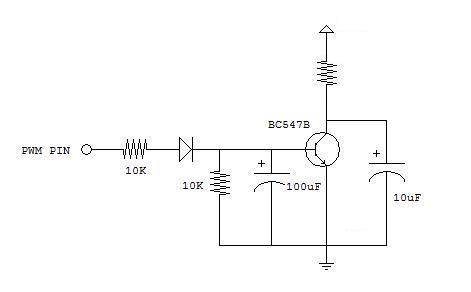# PWM driving a 0 - 2.5A Load

Hi all,

I am creating a load of 2.5A through high wattage resistors. I am driving it using a N channel MOSFET (IRL2910). I have a current shunt (0 - 3A, 0 - 75mA) in series with the load so I can monitor the current it is using. I would like to read the 0 - 75mA from the current shunt but it is quite noisy as expected due to the on/off of the FET. I have used a RC low pass filter but I cannot take a steady reading in Arduino even if I take 200 readings and average them out! Any ideas would be most appreciated, thanks.

An idea, post your code in code tags.

Another idea, post a schematic.

Is that 0 - 75 mA, or is it 0 - 75 mV?

The current shunt is essentially a small resistance of 0.025 ohms.
0.025ohms x 3amps=0.075 volts full scale. I am measuring across this small resistance. As PWM is controlling the current through a N channel mosfet, the voltage I am measuring across the shunt is quite noisy. This is reflected in the ADC being read into the arduino and being displayed on an LCD. The readings are unstable.

The code is just reading the signal on from the ADC pin.

As it's unlikely the signal will be smooth enough to get a steady reading on the ADC, does anyone have any suggestions?!! Thanks

You can use a moving average filter or a Simple Kalman Filter to add more signal smoothing.

What exactly is the purpose? You want to measure current?

Even a ACS712 would work instead of the current shunt.

Can you put ADC input signal snap of DSO?

Filtering a PWM signal can be done with something like thisOr even without the transistor you should be able to remove the ripple sufficiently to take average readings, and you won't need to tweak it at all. All you will have to do is take the voltage drop of the diode into account.

Pic showing output from voltage from current shunt. This is is reflecting the PWM signal driving the FET at around 50% duty cycle.

Yes, I would like to measure the current and read it into ADC and then dispay the current measurement on a LCD. The purpose is to measure vehicle damper current. The ACS712 looks interesting. Can't quite make out from the datasheet if the ACS712 can handle a 3A load? Is there a possiblility that it also will try to follow that same profile of the PWM signal? Or is it likely to average it out and give a stable DC output?

20 amps current

I always use a Kalman filter with A:D readings.

``````void fReadCurrent( void * parameter )
{
float ref_voltage = 3.3f;
float offSET = .0f;
uint64_t TimePastKalman  = esp_timer_get_time(); // used by the Kalman filter UpdateProcessNoise, time since last kalman calculation
SimpleKalmanFilter KF_I( 1.0f, 1.0f, .01f );
float mA = 0.0f;
int   printCount = 0;
const float mVperAmp = 100.0f;
float Voltage = 0;
float Power = 0.0;
String powerInfo = "";
powerInfo.reserve( 150 );
while ( !MQTTclient.connected() )
{
}
const TickType_t xFrequency = 1000; //delay for mS
for (;;)
{
Voltage = ( (adcValue * ref_voltage) / ADbits ) + offSET; // Gets you mV
mA = Voltage / mVperAmp; // get amps
KF_I.setProcessNoise( (esp_timer_get_time() - TimePastKalman) / 1000000.0f ); //get time, in microsecods, since last readings
mA = KF_I.updateEstimate( mA ); // apply simple Kalman filter
TimePastKalman = esp_timer_get_time(); // time of update complete
printCount++;
if ( printCount == 60 )
{
xSemaphoreTake( sema_CalculatedVoltage, portMAX_DELAY);
Power = CalculatedVoltage * mA;
//log_i( "Voltage=%f mA=%f Power=%f", CalculatedVoltage, mA, Power );
printCount = 0;
powerInfo.concat( String(CalculatedVoltage, 2) );
xSemaphoreGive( sema_CalculatedVoltage );
powerInfo.concat( ",");
powerInfo.concat( String(mA, 2) );
powerInfo.concat( ",");
powerInfo.concat( String(Power, 4) );
xSemaphoreTake( sema_MQTT_KeepAlive, portMAX_DELAY );
MQTTclient.publish( topicPower, powerInfo.c_str() );
xSemaphoreGive( sema_MQTT_KeepAlive );
powerInfo = "";
}
}
} //void fReadCurrent( void * parameter )
``````

Code to read the current sensor and uses a Kalman filter. Code is written for an ESP32 running under freeRTOS, the ESP32's built in OS. freeRTOS is great for a state machine or multi-threading or multi-processing.

Thanks ldahowalker, have you ever used a ACS712? Just wondering if it's worth giving one a try? I think it's got to be an improvement of what I currently (pardon the pun) have?

That code, from post #11, is a demo of the Kalman Filter using a ACS712.

A larger value for the current sense resistor would probably help.

I see. If I try it without the software filtering, do you think I will get a clean enough signal to read into a ADC? Just want the ripple effect minimsed.

I have found that readings from the A:D are better off filtered, software, which should not prevent you from rolling your own thing.

This topic was automatically closed 120 days after the last reply. New replies are no longer allowed.